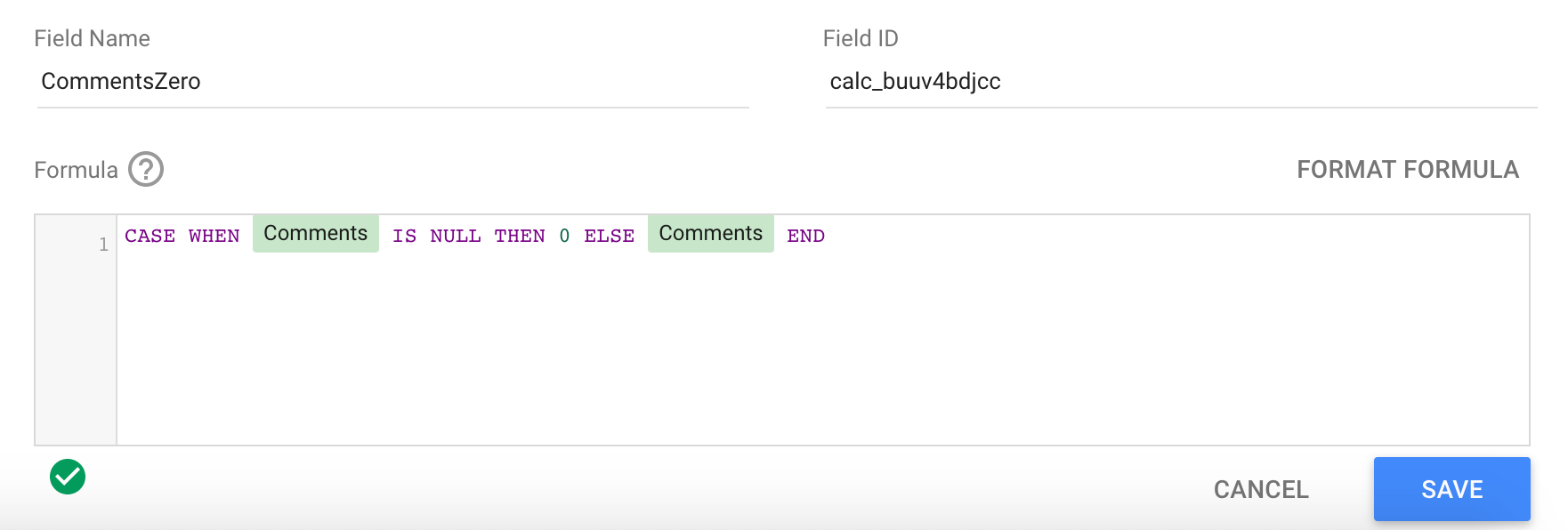# Converting Null Values to Zero

If you need the data for a field to be a zero instead of a null value, so that you use math functions on it properly, you can use calculated fields to convert it.

The basic formula is:

`CASE WHEN <yourfieldname> IS NULL THEN 0 ELSE <yourfieldname> END`Another option is to use the NARY_MAX function:

`NARY_MAX(<yourfieldname>,0)`

In this example, used with the Cost metric:This technique is necessary when using metrics to create custom calculations for your reports - if any value in the math is null, it will turn the whole result null. To get around this, you can create a new calculated field for each metric with the above formulas, and then use these fields instead to build your custom calculations. With the nulls as zeros, the math will be calculated correctly.

## If this does not work:

If you try this and still get "null" values or "0"s for unique count metrics that should have non-zero values (like Reach, views, etc.), you may be running into the "can't be calculated, would require summing deduplicated values" issue. Using the above method won't help with those, you need to use a different approach. Please see this guide for how to address that issue: Can't Be Calculated, Would Require Summing Deduplicated Values

## Cosmetic Zero

If you want to convert a value to "0" because it looks nicer in your report but don't plan to use it for calculations, you can also use a setting in Data Studio to change it to render as "0" (or other value to represent no data).

Click on the report object to update and then click the STYLE tab. Scroll down to the "Missing Data" section and adjust the value there.There's many options to choose from:Did you find it helpful? Yes No

Send feedback